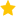See the live dashboard for CensusAtSchool 2023

This workshop uses hands-on learning experiences to explore probability distributions.  We trial some activities investigating: discrete and continuous probability distributions; mean and standard deviation of random variables; distribution of true probabilities versus distribution of model estimates of probabilities versus distribution of experimental estimates of probabilities.

• Probability Distributions , M. Steel

Resources

• Distributions Ppt
• Looking at distributions information for teachers on what is expected at Level 7 and 8 in terms of distribution shape, sample size and the context of the data.
• Teaching distributions xls a simulation of heights of men that explores the sampling variation and the effect of increasing sample size.

• Event: 2012 Statistics Teachers' Day
• Keywords: Discrete random variables, Expected values, Probability distributions
• (Last updated: 22/09/19. Added: 08/11/12)
• ## Rate this resource:(1 ratings)